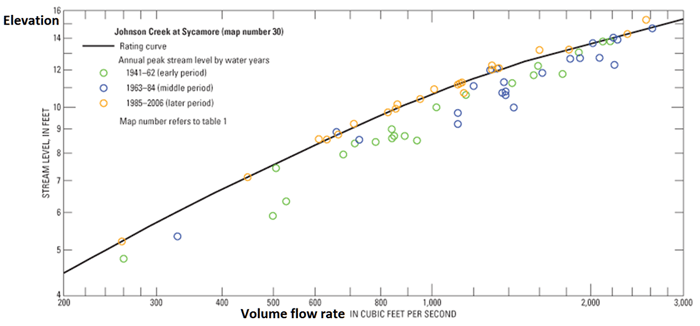# New Boundary Conditions

For numerical modeling of river flows, typically water elevation is required at the upstream boundary. Yet water elevation in natural environmental systems is often unknown and has to be estimated. Improper elevation estimation, however, can generate nonphysical results. In FLOW-3D v11.1, which has just been released, users now have the option of having boundary water elevations dynamically adapt to the conditions inside the domain. This can be achieved through the use of rating curves provided by the user, or in the absence of rating curves; the solver can dynamically adjust the elevation to vary smoothly with the conditions inside the fluid domain. These variations may be further constrained to certain Froude regimes or absolute elevation bounds.Figure 1. Rating curve for John Creek at Sycamore from USGS

## Rating curves

Rating curves define elevation variations at a given location in a river reach according to inflow rates at that location. A relationship between elevation and volume flow rate is established by physical measurements at a particular cross section of the river. Rating curves for rivers in the United States are available from the USGS (U. S. Geological Survey). A typical rating curve will have volume flow rate on the X-axis and elevation on the Y-axis (Figure 1).

## Natural inlets

In a case where inflow rate is known but a rating curve is unavailable, a natural boundary condition can be selected in the FLOW-3D model setup interface. At a given cross-section, for a certain specific energy, there can be two possible depths. This arises from the quadratic relationship between specific energy and the depth (see the equation below). The two mathematical depths manifest into supercritical and subcritical depths in reality. In the case of a perfect unique solution to the quadratic equation, the flow is critical.$E=\frac{{{{q}^{2}}}}{{2g{{y}^{2}}}}+y$

Here, E is the specific energy, q is the unit discharge, g is acceleration due to gravity and y is the height of fluid. Graphically, the specific energy and depth relationship can be seen in Figures 2-4.Figure 3. Possibility of two flow depths (supercritical and subcritical) for the same value of specific energyFigure 4. Flow depth can be critical (yc) for a unique value of depth and specific energy. In this case, flow is neither subcritical nor supercritical.

## Applying new boundary conditions

A rating curve can only be defined for volume flow rate and pressure boundary conditions in FLOW-3D v11.1. For volume flow rate type boundary conditions, instantaneous elevations are calculated using the rating curve to find the elevation corresponding to the flow rate. For a pressure type boundary condition, the volume flow rate is calculated by the solver and elevation is calculated using the rating curve. Rating curves can be applied at both upstream and downstream boundaries. It is important to note that an incorrect rating curve can result in nonphysical flow fluctuations.

Natural boundary conditions can only be defined at the inlet. Flow categories can be defined from one of the following:

• Supercritical flow (y<yc)
• Subcritical flow (y>yc)
• Critical flow (y=yc)
• Automatic flow regime (calculated by the solver)

The user can define maximum and minimum limits of elevation for any of these flows. If the depth for a particular flow regime violates the maximum and minimum limits of elevation, the latter will take precedence.

## Sample simulation results

Simulation 1 shows the river reach with a natural inlet under volume flow rate boundary condition at the left boundary and a rating curve for the outlet is defined as a pressure boundary condition at the right boundary.  The evolution of water elevation is shown for both upstream and downstream boundaries simultaneously. The simulation shows smooth variation of elevations at the boundaries without any fluctuations or nonphysical behavior. Therefore, this new development in FLOW-3D v11.1 allows for more natural variations of the water level for environmental applications.

Evolution of water elevation in a river reach with natural boundary condition at the inlet and a rating curve at the outlet.